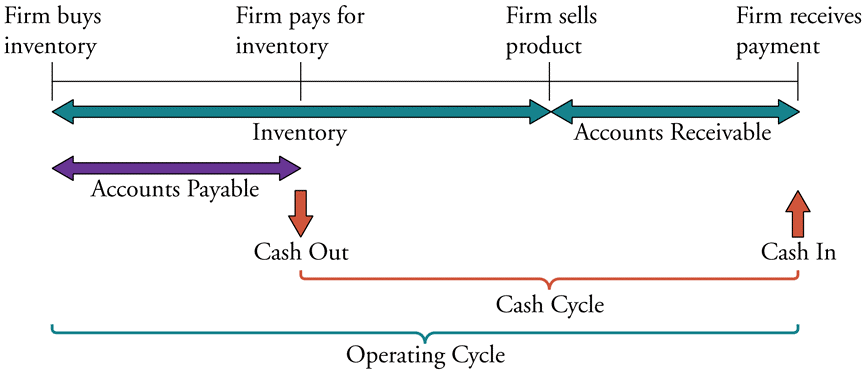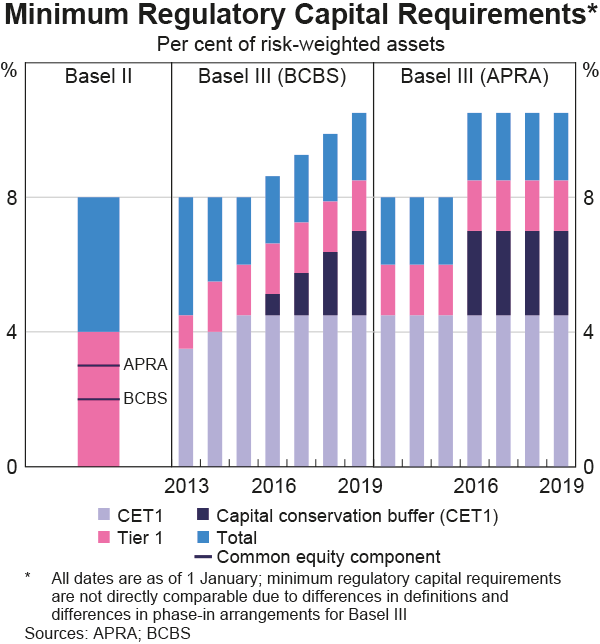# Fight Finance

#### CoursesTagsRandomAllRecentScores

A European bond paying annual coupons of 6% offers a yield of 10% pa.

Convert the yield into an effective monthly rate, an effective annual rate and an effective daily rate. Assume that there are 365 days in a year.

All answers are given in the same order:

$$r_\text{eff, monthly} , r_\text{eff, yearly} , r_\text{eff, daily}$$

The following is the Dividend Discount Model used to price stocks:

$$p_0=\frac{d_1}{r-g}$$

Which of the following statements about the Dividend Discount Model is NOT correct?

The CAPM can be used to find a business's expected opportunity cost of capital:

$$r_i=r_f+β_i (r_m-r_f)$$

What should be used as the risk free rate $r_f$?

The investment decision primarily affects which part of a business?

The below diagram shows a firm’s cash cycle.Which of the following statements about companies’ cash cycle is NOT correct?

The below graph from the RBA shows the phase-in of the Basel 3 minimum regulatory capital requirements under the Basel Committee on Banking Supervision (BCBS) on the left panel and in Australia under the Australian Prudential Regulatory Authority (APRA) on the right panel.Which of the following statements about the Basel 3 minimum regulatory capital requirements as at 2019 is NOT correct? All minimum amounts exclude the 2.5% counter-cyclical buffer.

The Basel 3 minimum regulatory capital requirement as a percent of Risk Weighted Assets (RWA) is:

A stock's returns are normally distributed with a mean of 8% pa and a standard deviation of 15 percentage points pa. What is the 99% confidence interval of returns over the next year? Note that the Z-statistic corresponding to a one-tail:

• 90% normal probability density function is 1.282.
• 95% normal probability density function is 1.645.
• 97.5% normal probability density function is 1.960.
• 99% normal probability density function is 2.326.
• 99.5% normal probability density function is 2.576

The 99% confidence interval of annual returns is between:

You are an equities analyst trying to value the equity of the Australian supermarket conglomerate Woolworths, with ticker WOW. In Australia, listed companies like Woolworths tend to pay dividends every 6 months. The payment around September is the final dividend and the payment around March is called the interim dividend. Both occur annually.

• Today is mid-November 2018.
• WOW's last final dividend of $0.50 was two months ago in mid-September 2018. • WOW's last interim dividend of$0.43 was eight months ago in mid-March 2018.
• Judging by the dividend history and WOW's prospects, you judge that the growth rate in the dividends will be 3% pa forever.
• Assume that WOW's total cost of equity is 6.5% pa. All rates are quoted as nominal effective rates.
• The dividends are nominal cash flows and the inflation rate is 2.5% pa.

What should be the current share price of WOW?

In an initial public offering (IPO):

Find the Macaulay duration of a 2 year 5% pa annual fixed coupon bond which has a \$100 face value and currently has a yield to maturity of 8% pa. The Macaulay duration is: